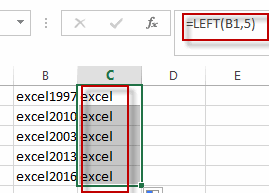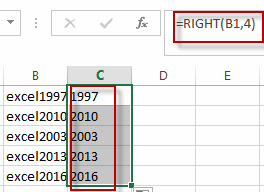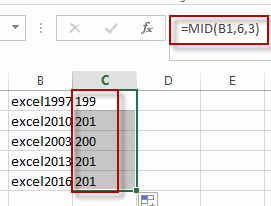# Extract Part of Text String

This post will guide you how to extract part of text string from a cell in Excel. How do I extract a word from a text string in Excel. How to extract a substring from a text string in a Cell in Excel. How to extract a substring from the most left of a text string in Excel. How to extract a word from the most right of a text string in Excel.

Assuming that you have a list of data in the range of cells B1:B5, and you want to get the most left 5 characters from cells, or you want to get the most right 4 characters as a substring from cells, how to achieve it. Let’s see the below detailed steps.

## Extract Word from the Left of a String

To extract word from the left of a text string in a cell in Excel, you can create a formula based on the LEFT function. Like this:

`=LEFT(B1,5)`

Type this formula into a blank cell, then press Enter key in your keyboard. And drag the AutoFill Handle over to other cells to apply this formula.

Let’s see the result:You will see that all the most left 5 characters have been extracted into the range C1:C5.

## Extract Word from the right of a String

If you want to extract a substring from the right of a text string in a cell, you can use a formula based on the RIGHT function. Like this:

`=RIGHT(B1,4)`

Type this formula in a blank cell, Press Enter key to apply this formula. Then drag the AutoFill handle over other cells.## Extract Word from the Mid of a String

If you want to extract a substring from the mid of a text string in a cell, you can use a formula based on the MID function. Like this:

`=MID(B1,6,3)`

This formula will extract a substring from a text string in Cell B1, and the starting position is 6, and the length of substring is 3.### Related Functions

• Excel LEFT function
The Excel LEFT function returns a substring (a specified number of the characters) from a text string, starting from the leftmost character.The LEFT function is a build-in function in Microsoft Excel and it is categorized as a Text Function.The syntax of the LEFT function is as below:= LEFT(text,[num_chars])…t)…
• Excel RIGHT function
The Excel RIGHT function returns a substring (a specified number of the characters) from a text string, starting from the rightmost character.The syntax of the RIGHT function is as below:= RIGHT (text,[num_chars])…
• Excel MID function
The Excel MID function returns a substring from a text string at the position that you specify.The syntax of the MID function is as below:= MID (text, start_num, num_chars)…